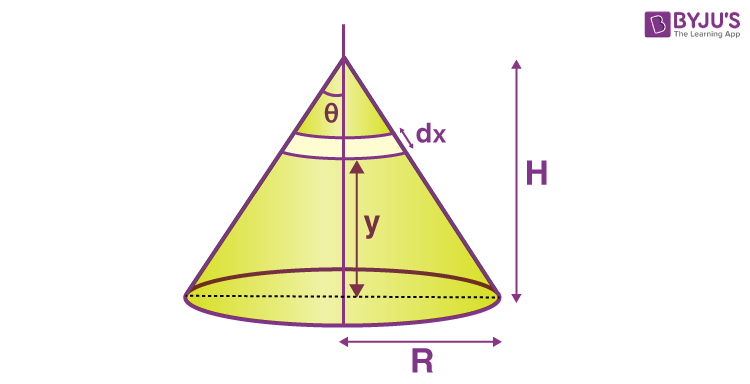Win up to 100% scholarship on Aakash BYJU'S JEE/NEET courses with ABNAT Win up to 100% scholarship on Aakash BYJU'S JEE/NEET courses with ABNAT

# Centre of Mass of Hollow Cone

Centre of mass is the point at which the entire mass of the object is concentrated. The cone can be either a solid cone or a hollow cone. The hollow cone will have the centre of mass at a distance of 2h/3 from the apex and at a distance of h/3 from the base.

Let us determine the value of the centre of mass (centre of gravity) of a hollow cylinder.Let us consider a hollow cone of height H. A circular ring of thickness dx is considered at a height y from the base of the cone. The radius of the ring is taken as x sinθ.

Area of the circular ring, dA = 2π(xsinθ)dx

Mass of the circular ring of thickness dx is,

$$\begin{array}{l}dM=\frac{M}{\pi R\sqrt{R^{2}+H^{2}}}\times 2\pi xsin\theta dx\end{array}$$
$$\begin{array}{l}dM = \frac{M}{R\sqrt{R^{2}+H^{2}}}\times 2x\frac{R}{\sqrt{R^{2}+H^{2}}}dx\end{array}$$

dM = 2Mxdx/(R2 +H2)….(1)

y = H – xcosθ

$$\begin{array}{l}y = H -\frac{xH}{\sqrt{H^{2}+R^{2}}}….(2)\end{array}$$

Centre of mass,

$$\begin{array}{l}C = \frac{\int ydM}{\int dM}….(3)\end{array}$$

Substitute the values from equa (1) and equa (2) in equa (3) and integrate it

$$\begin{array}{l}C =\frac{\int_{0}^{\sqrt{R^{2}+H^{2}}} H(1-\frac{x}{\sqrt{R^{2}+H^{2}}})\times \frac{2M}{R^{2}+H^{2}}xdx}{\int_{0}^{\sqrt{R^{2}+H^{2}}}\frac{2M}{R^{2}+H^{2}}xdx }\end{array}$$

Solving the above equation we get C = H/3

Centre of Mass of the hollow cone, C = H/3

Where H is the height of the cone.

## Centre of Mass and Collision## Centre of mass of a uniform solid cone## Frequently Asked Questions on Centre of Mass of Hollow Cone

Q1

### Where does the centre of mass of a hollow cone lie?

A hollow cone’s centre of mass is placed along a line. This line runs perpendicular to the base and ends at the apex. With respect to the apex, the centre of mass is 2/3 the height of the cone.

Q2

### What is the centre of mass?

The centre of mass is the point where the object’s entire mass is concentrated.

Q3

### What is the difference between the centre of mass and the centre of gravity?

Weight determines the centre of gravity, whereas mass determines the centre of mass. The two are equivalent when the gravitational field across an object is uniform.
In practice, the centre of gravity is the point at which an item can be properly balanced; the net torque due to gravity at that point is zero. The centre of mass, on the other hand, is the mass distribution’s average location. The object would spin around the centre of mass if it were given some angular momentum.

Test your Knowledge on Hollow cone